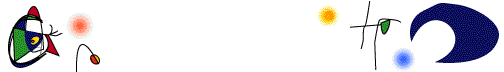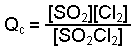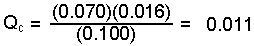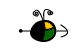Calculating the Reaction Quotient, Q

The expression for the reaction quotient, Q, looks like that used to calculate an equilibrium constant but Q can be calculated for any set of conditions, not just for equilibrium.

Q can be used to determine which direction a reaction will shift to reach equilibrium.  If K > Q, a reaction will proceed forward, converting reactants into products.  If K < Q, the reaction will proceed in the reverse direction, converting products into reactants.  If Q = K then the system is already at equilibrium.

In order to determine Q we need to know:

• the equation for the reaction, including the physical states,
• the quantities of each species (molarities and/or pressures), all measured at the same moment in time.
To calculate Q:
• Write the expression for the reaction quotient.
• Find the molar concentrations or partial pressures of each species involved.
• Subsitute values into the expression and solve.
Example:  0.035 moles of SO2, 0.500 moles of SO2Cl2, and 0.080 moles of Cl2 are combined in an evacuated 5.00 L flask and heated to 100oC.  What is Q before the reaction begins?  Which direction will the reaction proceed in order to establish equilibrium?

SO2Cl2(g)SO2(g) + Cl2(g)       Kc = 0.078 at 100oC

• Write the expression to find the reaction quotient, Q.• Since Kc is given, the amounts must be expressed as moles per liter (molarity).  The amounts are in moles so a conversion is required.

 0.500 mole SO2Cl2/5.00 L = 0.100 M SO2Cl2 0.035 mole SO2/5.00 L = 0.070 M SO2 0.080 mole Cl2/5.00 L = 0.016 M Cl2

• Substitute the values in to the expression and solve for Q.• Compare the answer to the value for the equilibrium constant and predict the shift.
0.078 (K) > 0.011 (Q)

Since K >Q, the reaction will proceed in the forward direction in order to increase the concentrations of both SO2 and Cl2 and decrease that of SO2Cl2 until Q = K.